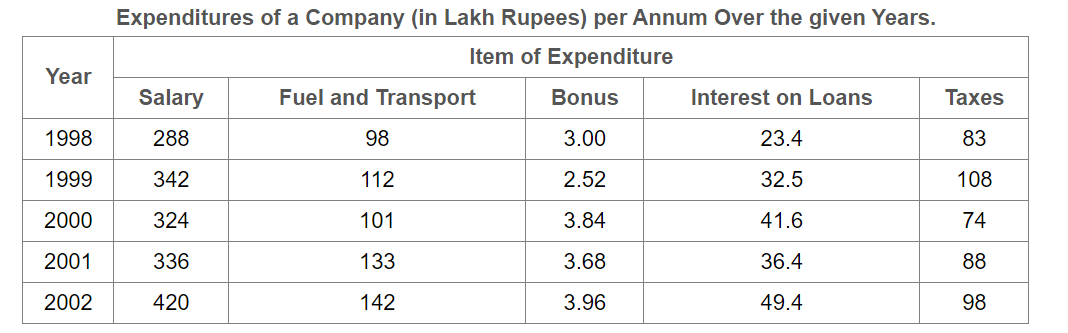# E-PolyLearning

 1. Study the following table and answer the question based on it. What is the average amount of interest per year which the company had to pay during this period?a. Rs. 32.43 lakhs b. Rs. 33.72 lakhs c. Rs. 34.18 lakhs d. Rs. 36.66 lakhs

 2. Study the following table and answer the question based on it. The total amount of bonus paid by the company during the given period is approximately what percent of the total amount of salary paid during this period?a. 0.1% b. 0.5% c. 1% d. 1.25%
 3. Study the following table and answer the question based on it. Total expenditure on all these items in 1998 was approximately what percent of the total expenditure in 2002?a. 62% b. 66% c. 69% d. 71%
 4. Study the following table and answer the question based on it. The total expenditure of the company over these items during the year 2000 is?a. Rs. 544.44 lakhs b. Rs. 501.11 lakhs c. Rs. 446.46 lakhs d. Rs. 478.87 lakhs
 5. Study the following table and answer the question based on it. The ratio between the total expenditure on Taxes for all the years and the total expenditure on Fuel and Transport for all the years respectively is approximately?a. 4:7 b. 10:13 c. 15:18 d. 5:8